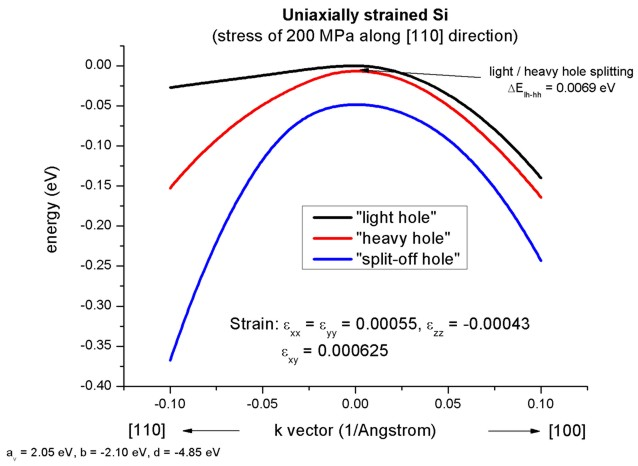nextnano.com  nextnano³  Download | Search | Copyright | Publications  * password protected nextnano³ software1D strained silicon

# nextnano3 - Tutorial

## k.p dispersion in bulk, biaxially strained and uniaxially strained Si

Author: Stefan Birner

If you want to obtain the input files that are used within this tutorial, please check if you can find them in the installation directory.
If you cannot find them, please submit a Support Ticket.
```-> 1Dbulk_kp_dispersion_Si.in                               - ```(unstrained)``` -> 1Dbulk_kp_dispersion_Si_3Dplot.in                        - ```(unstrained)``` -> 1Dbulk_kp_dispersion_strained_Si.in                      - ```(biaxially strained)``` -> 1Dbulk_kp_dispersion_strained_Si_3Dplot.in               - ```(biaxially strained)``` -> 1Dbulk_kp_dispersion_uniaxial_strained_Si_read.in        - ```(uniaxially strained)``` -> 1Dbulk_kp_dispersion_uniaxial_strained_Si_3Dplot_read.in - ```(uniaxially strained)``` ```Uniaxial strain file to be read in:``` strain_cr1D_read_in_uniaxial110.dat  ```

## Band structure of bulk Si

• We want to calculate the dispersion E(k) from |k|=0 nm`-`1 to |k|=0.1 nm`-`1 along the following directions in k space:
-  to  where  is the Gamma point.
-  to 
We compare 6-band k.p theory results vs. single-band (effective-mass) results.
• We calculate E(k) for bulk Si (unstrained).

### Bulk dispersion along  and 

`-> 1Dbulk_kp_dispersion_Si.in`

• ``` \$output-kp-data  destination-directory    = kp/  bulk-kp-dispersion       = yes  grid-position            = 2d0                      ! ``` in units of``` [nm]  !----------------------------------------  ! Dispersion along  direction  ! Dispersion along  direction  ! maximum |k| vector = 1.0 [1/nm]  !----------------------------------------  k-direction-from-k-point = 0.7071d0  0.7071d0  0d0 ! ```k-direction and range for dispersion plot``` [1/nm] ```The maximum value of |k| is SQRT(0.7071² + 0.7071²) = 0.1` [1/nm]`.```  k-direction-to-k-point   = 1.0d0     0d0       0d0 ! ```k-direction and range for dispersion plot``` [1/nm]  number-of-k-points       = 100                     ! ```number of k points to be calculated (resolution)
``` shift-holes-to-zero      = yes                     ! 'yes' ```or``` 'no' \$end_output-kp-data```
• We calculate the pure bulk dispersion at` grid-position = 2d0`, i.e. for the material located at the grid point at 2 nm. In our case this is Si but it could be any strained alloy. In the latter case, the k.p Bir-Pikus strain Hamiltonian will be diagonalized.
The grid point at` grid-position `must be located inside a quantum cluster.
`shift-holes-to-zero = yes `forces the top of the valence band to be located at 0 eV.
How often the bulk k.p Hamiltonian should be solved can be specified via` number-of-k-points`. To increase the resolution, just increase this number.
• Start the calculation.
The results can be found in:```  kp_bulk/bulk_6x6kp_dispersion_as_in_inputfile_kxkykz_000_kxkykz.dat ``` (6-band k.p)
`  kp_bulk/bulk_sg_dispersion.dat                                      `(single-band approximation)
• Note that for values of |k| larger than 0.1, k.p theory might not be a good approximation any more.

### Step 2: Plotting E(k)

• Here we will have to visualize the results of both Step 1 and Step 2.
• The final figure will look like this:
(In silicon, the directions , , , [-100], [0-10] and [00-1] are equivalent in the unstrained case
and so are the directions , , , [-110], [-101], [0-11], [1-10], [10-1], [01-1], [-1-10], [-10-1] and [0-1-1].)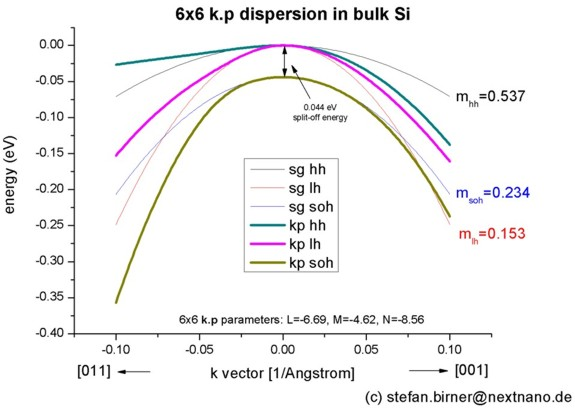The split-off energy of 0.044 eV is identical to the split-off energy as defined in the database:
```  6x6-kp-parameters = ... 0.044d0 ```In the unstrained case, the light hole seems to be kind of isotropic in contrast to the heavy and split-off hole which clearly exhibit anisotropy. The light hole "isotropy" can be checked further below in the 2D and 3D plots of the dispersion curves.
``` ```For comparison, the single-band (effective-mass) dispersion is also shown. It corresponds to the following effective hole masses:
``` valence-band-masses = 0.537d0 0.537d0 0.537d0 ! [m0] ```heavy hole```                        0.153d0 0.153d0 0.153d0 ! [m0] ```light hole```                        0.234d0 0.234d0 0.234d0 ! [m0] ```split-off hole``` ```(The effective mass parameters were taken from K.W. Boer, Survey of Semiconductor Physics, Vol. 2 (1990).)
The effective mass approximation is a simple parabolic dispersion which is isotropic (i.e. no dependence on the k vector direction).

One can see that for |k| < 0.1 [1/nm] the single-band approximation is in good agreement with 6-band k.p but differ at larger |k| values substantially.

Plotting E(k) in three dimensions

`-> 1Dbulk_kp_dispersion_Si_3Dplot.in`

Alternatively one can print out the 3D data field of the bulk E(k) = E(kx,ky,kz) dispersion.

``` \$output-kp-data  ...  bulk-kp-dispersion-3D = yes  grid-position         = 2d0              ! ```in units of``` [nm] !----------------------------------------! maximum |k| vector = 1.0 [1/nm]! maximum |k| vector = 1.5 [1/nm]!----------------------------------------! ```Note: The 3D and 1D plots in the tutorial use` "1.0d0"`, the 2D plots use` "1.5d0"`.```  k-direction-to-k-point = 1.0d0 0d0 0d0   ! ```(3D plot) k-direction and range for dispersion plot``` [1/nm]! k-direction-to-k-point = 1.5d0 0d0 0d0   ! ```(2D plot) k-direction and range for dispersion plot``` [1/nm]   number-of-k-points    = 40               ! ```number of k points to calculated (resolution)```  shift-holes-to-zero   = yes              ! 'yes' ```or``` 'no' ```
The meaning of` number-of-k-points = 40` is the following:
40 k points from '```- maximum |k| vector' ```to zero (plus the Gamma point) and
40 k points from zero to  '`+ maximum |k| vector'` (plus the Gamma point) along all three directions,
i.e. the whole 3D volume then contains 81 * 81 * 81 = 531441 k points.

The following figure shows the constant energy surface at E = 25 meV below the valence band edge for the heavy hole (left) and for the light hole (right).
In the unstrained case, both heavy and light hole are degenerate at k = 0. The color bars on the left side of each plot correspond to the 2D slices in these pictures.  The units are [eV].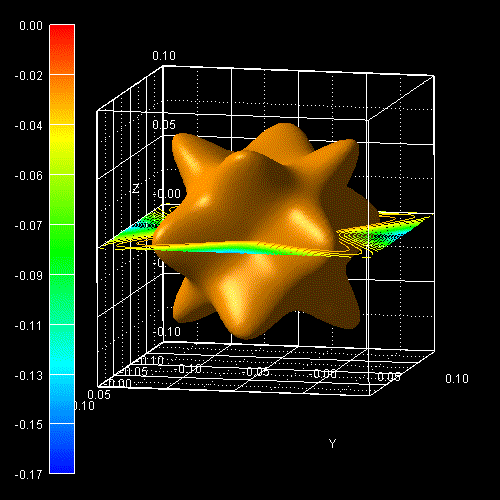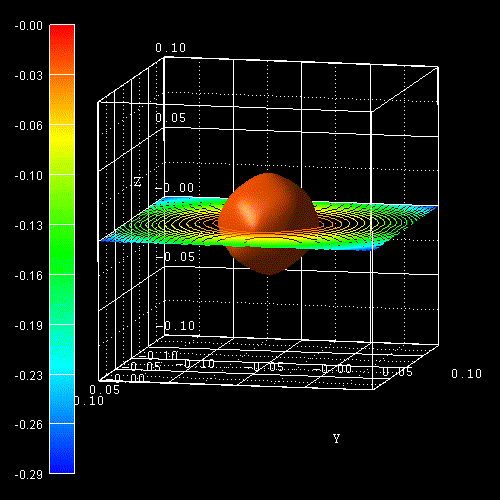The following figure shows the constant energy contours in the (kx,ky) plane of a 2D slice through the heavy hole (left) and the light hole (right) 3D E(kx,ky,kz) dispersion at kz = 0, i.e. E(kx,ky,0). The light hole is much more isotropic than the heavy hole.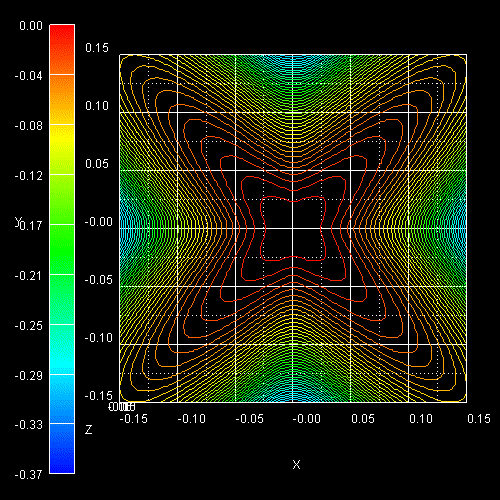These two figures are in very good agreement with the figures in the following paper:

Key Differences For Process-induced Uniaxial vs. Substrate-induced Biaxial Stressed Si and Ge Channel MOSFETs

S.E. Thompson, G. Sun, K. Wu, J. Lim, T. Nishida
Proc. IEEE-IEDM (International Electron Devices Meeting), 221 (2004)

Note that the figures in the paper were calculated using the empirical pseudopotentials method whereas ours are based on a 6-band k.p Hamiltonian.

Technical details: For the 3D plots we calculated the E(k) dispersion until a maximum k vector of kx = ky = kz = 1.0 nm`-`1 (`k-direction-to-k-point = 1.0d0 0d0 0d0`) whereas for the 2D plots we used a maximum k vector of kx = ky = kz = 1.5 nm`-`1 (```k-direction-to-k-point = 1.5d0 0d0 0d0```) as was done in the figures in the paper of S.E. Thompson et al. Thus the color bars at the left side of the figures differ between 2D and 3D.

## Band structure of strained Si

• Now we perform these calculations again for Si that is biaxially strained with respect to Si0.83Ge0.17. The Si0.83Ge0.17 lattice constant is larger than the Si one, thus Si is strained tensilely.
• The changes that we have to make in the input file are the following:

``` \$simulation-flow-control  ...  strain-calculation  = homogeneous-strain \$end_simulation-flow-control ```
``` \$domain-coordinates  ...  pseudomorphic-on    = Si(1-x)Ge(x)  alloy-concentration = 0.17d0 \$end_domain-coordinates ```As substrate material we take (relaxed) Si0.83Ge0.17 and assume that Si is strained pseudomorphically (`homogeneous-strain`) with respect to this substrate, i.e. Si is subject to a biaxial tensile strain.
• ```-> 1Dbulk_kp_dispersion_strained_Si.in ```
The figure shows the E(k) dispersion along the  and  (=) directions for biaxially, tensilely strained silicon. Note that the heavy and light holes are now "mixed" and not any longer "purely" heavy and light hole states. At the zone center (k = 0), the highest valence band has light hole character.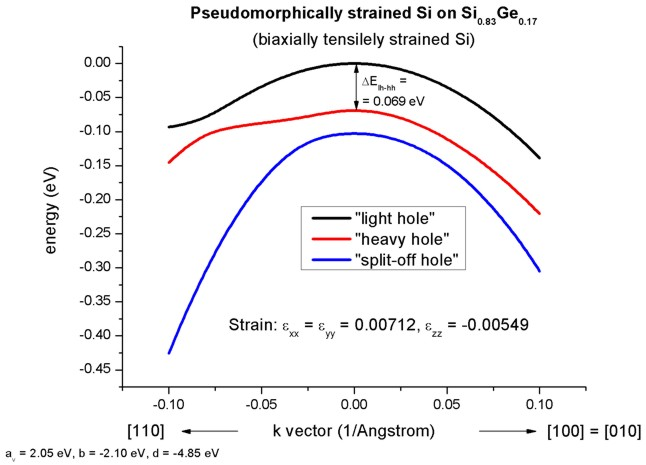(In silicon, the directions , , [-100] and [0-10] are equivalent in the biaxially strained case if the biaxial
stress is directed along the , [-100],  and [0-10] directions.
The directions , [-110], [1-10] and [-1-10] are also equivalent.)
• Due to the positive hydrostatic strain (i.e. increase in volume or negative hydrostatic pressure) we obtain a reduced band gap with respect to the unstrained Si (not shown in the figure).
Furthermore, the degeneracy of the heavy and light hole at k=0 is lifted by 69 meV and the light hole has been shifted above the heavy hole.
Now, the anisotropy of the holes along the different directions  and  is very pronounced. There is even a band anti-crossing along .

This biaxial strain can be expressed as applied stress (sigmaij) in units of GPa. The elastic constant of Si are:
```  elastic-constants = 165.77d0 63.93d0 79.62d0 ! c11,c12,c44 [GPa] ```298 K [Landolt-Boernstein]``` => ```sigmazz = 2 * c12 * e|| + c11 * e_|_ = 0   (constraint for pseudomorphic growth condition)
`=> `sigmaxx = sigmayy = (c11 + c12 ) * e|| + c12 * e|| = 1.2845 GPa
(e|| =  exx =  eyy  ;   e_|_ = ezz)
• Note that in the strained case, the effective-mass approximation is very poor.
• `-> 1Dbulk_kp_dispersion_strained_Si_3Dplot.in`

The following figure shows the constant energy contours in the (kx,ky) plane of a 2D slice through the ground state hole (left) and the first excited hole state (right) 3D E(kx,ky,kz) dispersion at kz = 0, i.e. E(kx,ky,0).

At the left figure one can see that around k = 0, the highest hole state has "light" hole character and for larger k values the "heavy" hole character dominates.
At the right figure, the second highest hole state is shown which is separated by 0.069 eV from the "light" hole (valence band edge). Around k = 0 it has heavy hole character and at larger k values it has "light" hole character as can be understood by comparing these plots to the unstrained dispersion curves.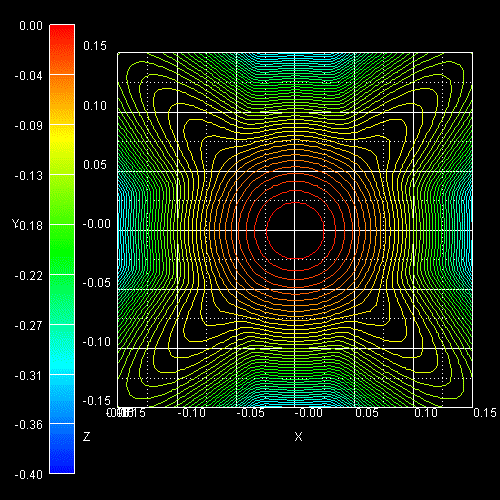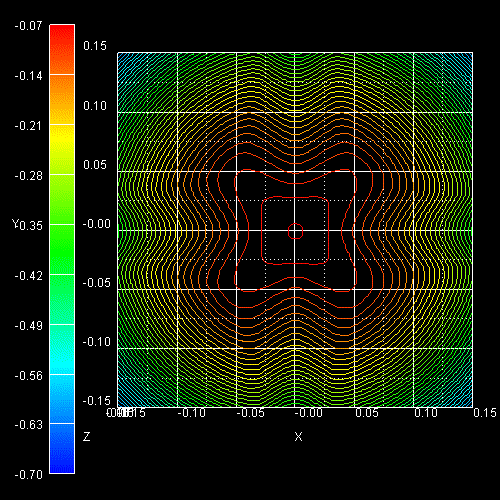The following figure shows the constant energy surface at E = 25 meV below the valence band edge for the ground state hole (left) and the constant energy surface at 94 meV below the valence band edge for the first excited hole state (right) (69 meV + 25 meV = 94 meV).
The degeneracy of heavy and light hole at k = 0 is lifted by 69 meV. The color bars on the left side of each plot correspond to the 2D slices in these pictures. The units are [eV].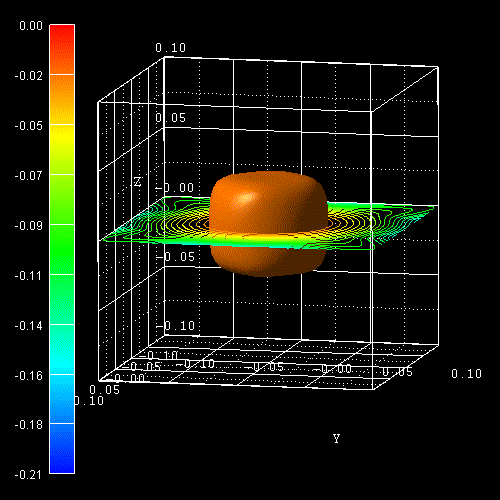## Reading in arbitrary strain tensors

Sometimes the user might want to specify an arbitrary strain tensor as input, read it in and calculate the relevant E(k) dispersion.
The procedure to do this is the following:

• ``` \$simulation-flow-control  ...  strain-calculation = import-strain-crystal-coordinate-system ```
``` \$import-data-on-material-grid  source-directory = strain/  filename-strain  = strain_cr1D_read_in_uniaxial110.dat \$end_import-data-on-material-grid```

The file``` strain/strain_cr1D_read_in_uniaxial110.dat ```must contain the strain tensor in the following format:
```   coordinate   exx  eyy  ezz  exy  exz  eyz ```The units for` coordinate `are [nm]. The strain tensor units are dimensionless` [-]`.
The strain tensor refers to the crystal coordinate system in this example.

Note: The` eij `components refer to shear strain and not to "engineer shear strain".
Shear strain is the average of two strain tensor components, i.e. ``` eij ```= 1/2 (dui/dxj + duj/dxi)  whereas engineer shear strain is defined as the total shear strain `eij `= dui/dxj + duj/dxi.

We read in the following strain tensor which corresponds to a small uniaxial tensile strain along the  direction (corresponding to a  stress of``` sigma = ```200 MPa):
```  exx = eyy = 0.00055   ezz = -0.00043   exy =  0.000625 ```It can be calculated by using these formulas where` S``ij```` ```is the contracted notation of the forth-rank compliance tensor` S``ijkl`:```  exx = eyy = (S11 + S12) * sigma / 2 = (7.681 + (-2.138)) [1/TPa] * 200 [MPa] / 2 = 0.00055  ezz = S12 * sigma = -2.138 [1/TPa] * 200 [MPa] = -0.00043  exy = S44 * sigma / 2 ```(engineer shear strain!!!)``` = 12.56 [1/TPa] * 200 [MPa] / 2 = 0.001256 ```To convert the "engineer shear strain definition" into the strain tensor definition used within nextnano³, one has to divide``` exy ```by a factor of` 2 `and thus one obtains``` exy =  0.000628```, i.e. the equation to be used reads:
``` exy = S44 * sigma / 4                   = 12.56 [1/TPa] * 200 [MPa] / 4 = 0.000628 ```This only applies to the offidagonal components``` eij  ```of the strain tensor.``` ```The elastic constants` c``ij```` ```can be converted into``` Sij ```by using the following formulas (P. Y. Yu, M. Cardona, "Fundamentals of Semiconductors, 3rd ed., p.140)```   c44 = 1/S44     => S44 = 1/c44 = 1/79.62 [GPa] = 12.56    [1/TPa] ```(calculated from elastic constants as given in nextnano³ database)```                                               = 12.70(9) [1/TPa] ```(Ref.: 4.4 Dielectrics and Electrooptics, Springer Online)```   c11 -  c12 = 1 / (S11 -  S12) = 165.77 -     63.93 = 101.84 [GPa]   c11 + 2c12 = 1 / (S11 + 2S12) = 165.77 + 2 * 63.93 = 293.63 [GPa]   => S11 =  7.681   [1/TPa] ```(calculated from elastic constants as given in nextnano³ database)```          =  7.73(8) [1/TPa] ``` (Ref.: 4.4 Dielectrics and Electrooptics, Springer Online)```   => S12 = -2.138   [1/TPa] ```(calculated from elastic constants as given in nextnano³ database)```          = −2.15(4) [1/TPa] ``` (Ref.: 4.4 Dielectrics and Electrooptics, Springer Online)``` ```The left figure shows the constant energy surface at E = 5 meV below the valence band edge for the ground state hole.
The color bars on the left side of each plot correspond to the 2D slices in these pictures.  The units are [eV].

`-> 1Dbulk_kp_dispersion_uniaxial_strained_Si_3Dplot_read.in`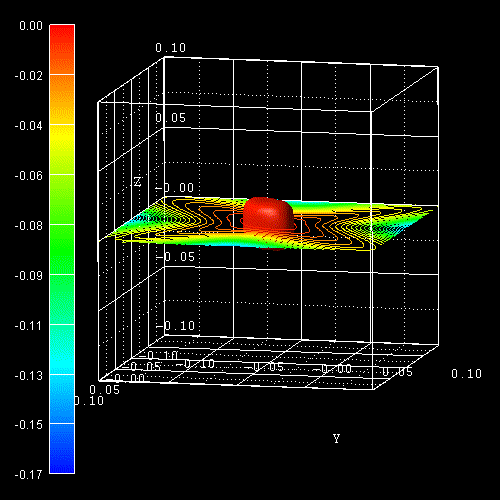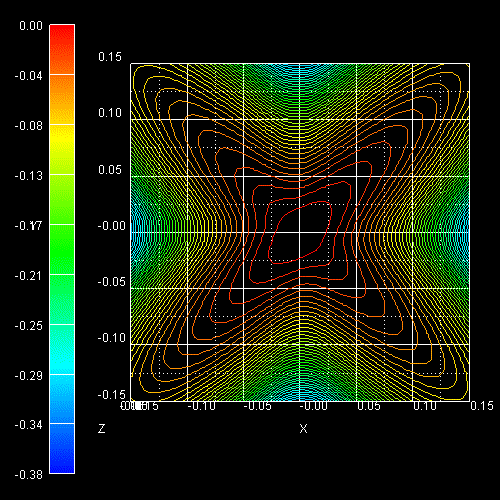This figure shows the E(k) dispersion along the lines from zero to  and from  to zero. Note that the directions  and [-110] are no longer equivalent as can be seen in the 2D plot shown above.

```-> 1Dbulk_kp_dispersion_uniaxial_strained_Si_read.in ```